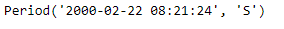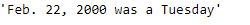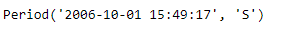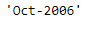Python | Pandas Period.strftime

• Last Updated : 06 Jan, 2019

Python is a great language for doing data analysis, primarily because of the fantastic ecosystem of data-centric python packages. Pandas is one of those packages and makes importing and analyzing data much easier.

Pandas Period.strftime() function returns the string representation of the Period, depending on the selected format. format must be a string containing one or several directives. The method recognizes the same directives as the time.strftime() function of the standard Python distribution, as well as the specific additional directives %f, %F, %q. (formatting & docs originally from scikits.timeries)

Syntax : Period.strftime()

Parameters : format

Return : string

Example #1: Use Period.strftime() function to return the value of the given period in a specified format.

 # importing pandas as pdimport pandas as pd  # Create the Period objectprd = pd.Period(freq ='S', year = 2000, month = 2, day = 22,                         hour = 8, minute = 21, second = 24)  # Print the Period objectprint(prd)

Output :Now we will use the Period.strftime() function to return the given period in (‘%b. %d, %Y was a %A’) format.

Note : '%b' is month name, %d is day of the month, %Y is year and %A is weekday name.

 # return the period in specified format.prd.strftime('% b. % d, % Y was a % A')

Output :As we can see in the output, the Period.strftime() function has returned the value of the given period in the specified format.

Example #2: Use Period.strftime() function to return the value of the given period in a specified format.

 # importing pandas as pdimport pandas as pd  # Create the Period objectprd = pd.Period(freq ='S', year = 2006, month = 10,                hour = 15, minute = 49, second = 17)  # Print the objectprint(prd)

Output :Now we will use the Period.strftime() function to return the given period in (‘%b-%Y’) format.

Note : '%b' is month name, %Y is year

 # return the period in specified format.prd.strftime('% b-% Y')

Output :As we can see in the output, the Period.strftime() function has returned the value of the given period in the specified format.

My Personal Notes arrow_drop_up